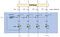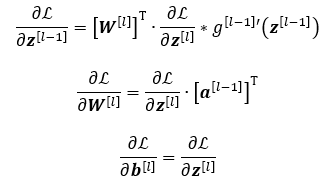# Transformer Networks: A mathematical explanation why scaling the dot products leads to more stable gradients

## How a small detail can make a huge difference

The main purpose of the self-attention mechanism used in transformer networks is to generate word embeddings which take the context of the surrounding words into account. The self-attention mechanism accomplishes this task by comparing every word in the sentence to every other word in the sentence and by subsequently combining contextually related words together.Computing the self-attention scores for the first word (Image by author)

The self-attention mechanism first computes three vectors (query, key and value) for each word in the sentence. To find contextually related words for a chosen word, we take the dot products of its query vector with the key vectors of every other word in the sentence…

# Derivative of the Softmax Function and the Categorical Cross-Entropy Loss

## A simple and quick derivation

In this short post, we are going to compute the Jacobian matrix of the softmax function. By applying an elegant computational trick, we will make the derivation super short. Using the obtained Jacobian matrix, we will then compute the gradient of the categorical cross-entropy loss.

# Softmax Function

The main purpose of the softmax function is to grab a vector of arbitrary real numbers and turn it into probabilities:

The exponential function in the formula above ensures that the obtained values are non-negative. Due to the normalization term in the denominator the obtained values sum to 1. Furthermore, all values lie between 0…

# Aleatory Overfitting vs. Epistemic Overfitting

## Approaching the two reasons why your model is not able to generalize well

If you've ever encountered the following problem:

I’m training a neural network and the training loss decreases, but the validation loss increases from the first epoch on. How can I fix this?

then you definitely should read this post to gain more understanding for the main two reasons why your model doesn’t generalize well.

# Aleatory Uncertainty

Let us start with the overfitting most machine learning practitioners are familiar with: overfitting caused by aleatory uncertainty, simply said overfitting caused by noisy data.

Here we have to deal with the fact, that the process generating real data, oftentimes, exhibits intrinsic randomness. Let us illustrate…

# Deriving the Backpropagation Equations from Scratch (Part 2)

## Gaining more insight into how neural networks are trained

In this short series of two posts, we will derive from scratch the three famous backpropagation equations for fully-connected (dense) layers:In the last post we have developed an intuition about backpropagation and have introduced the extended chain rule. In this post we will apply the chain rule to derive the equations above.

# Backpropagating the Error

Backpropagation starts in the last layer 𝐿 and successively moves back one layer at a time. For each visited layer it computes the so called error:

# Drawing the Transformer Network from Scratch (Part 1)

## Getting a mental model of the Transformer in a playful way

The Transformer Neural Networks — usually just called “Transformers” — were introduced by a Google-led team in 2017 in a paper titled “Attention Is All You Need”. They were refined and popularized by many people in the following work.

Like many models invented before it, the Transformer has an encoder-decoder architecture. In this post, we put our focus on the encoder part. We will successively draw all its parts in a Bottom-top fashion. Doing so will hopefully allow the readers to easily develop a “mental model” of the Transformer.

The animation below shows in fast motion what we will cover…

# Deriving the Backpropagation Equations from Scratch (Part 1)

## Gaining more insight into how neural networks are trained

In this short series of two posts, we will derive from scratch the three famous backpropagation equations for fully-connected (dense) layers:All following explanations assume we are feeding only one training sample to the network. How to extend the formulas to a mini-batch will be explained at the end of this post.

# Forward Propagation

We start with a short recap of the forward propagation for a single layer (in matrix form):

# How to efficiently implement Area Under Precision-Recall Curve (PR-AUC)

## How few lines of code can do real magic

This post is based on the implementation of PR-AUC published by Facebook AI Research in the scope of the Detectron project. It took me a while to understand how only a few lines of code are able to perform such a complicated task. Let me share my insights.

In the last post, we covered the theoretical background of PR-AUC. In this post, we will deepen our understanding by dissecting an efficient PR-AUC implementation. If you are not yet fully familiar with the notions of precision, recall, TP, FP etc. please revisit the last post.

We will again use an over-simplified…

# Gaining an intuitive understanding of Precision, Recall and Area Under Curve

## A friendly approach to understanding precision and recall

In this post, we will first explain the notions of precision and recall. We will try not to just throw a formula at you, but will instead use a more visual approach. This way we hope to create a more intuitive understanding of both notions and provide a nice mnemonic-trick for never forgetting them again. We will conclude the post with the explanation of precision-recall curves and the meaning of area under the curve. The post is meant both for beginners and advanced machine learning practitioners, who want to refresh their understanding. …## Thomas Kurbiel

Advanced Computer Vision & AI Research Engineer at APTIV Germany